Latest Banking jobs   »

# Quantitative Aptitude Quiz For Bank Foundation 2023 -22nd May

Directions (1-5): Two equations I and II are given below in each question. You have to solve these equations and give answer if
(a) if x<y
(b) if x> y
(c) if x≤ y
(d) if x≥ y
(e) if x= y or no relation can be established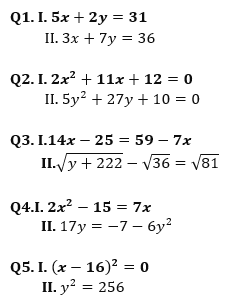Directions (6-10): In the given questions, two equations (I) & (II) are given. You have to solve both the equations and mark the answer accordingly.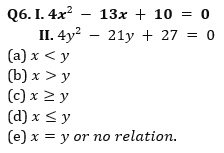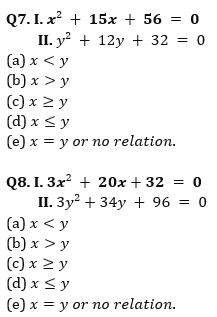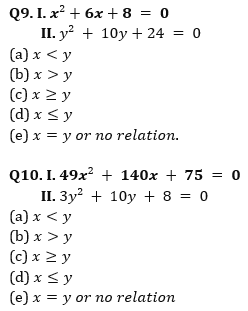Solutions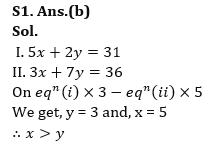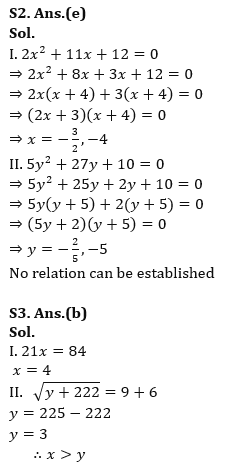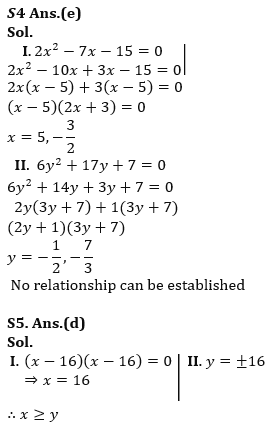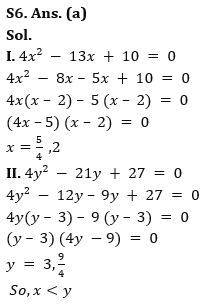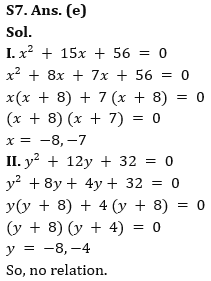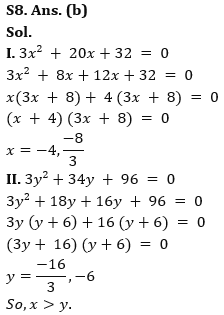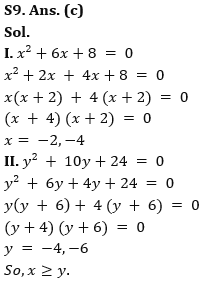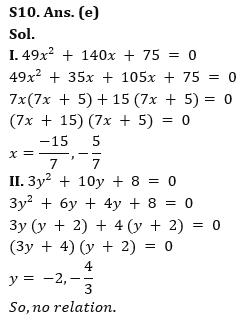.                                                                                                                   .                                                                                                                                                                 .                    .

## FAQs

### What is the selection process of the Bank Clerk?

The selection process of the Bank Clerk is Prelims & Mains.

#### Congratulations!Union Budget 2023-24: Free PDF